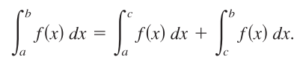The additive interval property (sometimes called the additive integral property) tells us that we can add up parts of an integral to get a whole.

Formally, the rule is stated as :All this is saying is that a definite integral (i.e. an area under a curve) from point a to point b can be split at a point “c“. The two pieces or “step integrals” can be added together to get the total area. More specifically, a definite integral evaluated over the closed interval [a, b] is the same as the sum of parts of the integrals (split at point c) over [a, c] and [c, b] when a ≤ c ≤ b.

The integral can be split at any point, and you can have more than two pieces.

## Example of the Additive Interval Property

The above image shows the definite integral of f(x) = 2x on the interval [0, 3]. Calculating this definite integral using the integral power rule, we get:We can split the integral at x = 2 and get the same result:## Using the Additive Property for Integrals

The addition problem can be useful to work backwards, finding intervals that have a certain area. For example, if you knew that the whole integral had an area of 9, and one part of the integral had an area of 3, you could conclude that the “missing” interval had an area of 6. From there, you could work out the missing bounds of integration.

## References

 Larson, R. & Edwards, B. (2017). Calculus, 11th Edition. Cengage Learning.

CITE THIS AS:
Stephanie Glen. "Additive Interval Property" From StatisticsHowTo.com: Elementary Statistics for the rest of us! https://www.statisticshowto.com/additive-interval-property/